# Daily Archives: February 8, 2016

## Read and Write and DIFFERENTIATE by printing – for Valentine’s Day!A significant part of my support role to kindergarten and primary teachers in recent years has been to provide instruction and / or resources to differentiate instruction for little people in literacy.

Within one class, the range of abilities can span four grades. A little one’s tolerance to frustration towards a task that is too difficult, or another’s need for something to challenge his or her learning for just the right amount of time, can be a tough order to fill – especially at the same time – after gym and snack time and before recess, library and reading buddies.

I have designed my Read and Write and More packages to allow teachers to #DifferentiateByPrinting. There are always activities in each of these products to address a variety of needs.

Valentine Scrambled Sentences:

(Note: The colored sentence strips shown in the first example are provided in the Valentine’s Day Read and Write and More set. There are two different sentence options to choose from to photocopy onto each color paper, with six identical scrambled sentences on each strip).

Having the option to place and paste sentence strips, place and copy sentence strips, work without the strips on easier or more challenging sentences, means that many pre-writers, early writers and / or are independent writers have an accessible starting point.

Valentine Rhyme and Ending Sounds:

See it, hear it, read it? Assessment or  fun practice activities?
I use the following pages for fun small group review, then send them home for the fridge, where my wish is for the back-of-the-cereal box effect.

When I am doing the following rhyme activity with students, I am mindful of my data. Those who need more practice listening for rhyme and ending sounds and who automatically compare letter patterns at the ends of words will get the sheet without print. Proficient rhymers who need more practice with looking closely at print will get the copy with the words. And, of course, other days there will be other activities for those who do not fit neatly into those categories.

Check out these fantastic ideas and resources on our Rhyme Time board below:

Valentine’s Day Read and Write and More contains a number of other fun literacy activities, including:-> Graphic Organizers, Writing Paper, B & W Cards to color with insert ->And for another day… …activities for the 100th day of school!

The Read and Write and More sets always contain three-part graphic organizers, thematic writing paper, and fun, literacy activities with an explanation page, ideas and options to help make activities accessible to a wide range of kindergarten to grade 2 students.

We all remember our little students on Valentine’s Day. Check out the freebies below to be sure no one is missed!Happy Valentine’s Week, my friends!

Save with Bundles!

## February Teacher Talk

Posted by Deann Marin of Socrates Lantern

Welcome to our February Teacher Talk.  All of us from the Teacher Talk collaborative would like wish you a Happy Valentines Day.  We have so many fab things this month from  proof-reading ideas, to ELA to math activities, to celebrating Black History Month and President’s Day, you don’t want to miss reading these blog posts from some awesome educators.If you’re interested in joining this unique group of teacher entrepreneurs and blogging buddies and our blog linky, sign up here….The Best of Teacher Entrepreneurs Marketing Cooperative. If you decide to join, be sure to mention one of our names.

<<<<<<<<<<<<<<<<<<<<<<<<<<<<<<<<<<<<<<<<<<<<<<<<<<<<<<<<<<<

The Proof is in the Pudding, Proof-Reading That Is!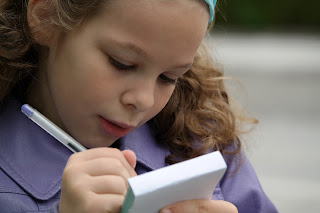As a veteran English and Social Studies teacher, I’d like to share some effective proof-reading tips that I’ve used to help students improve their writing skills, and to make my life a bit easier.

<<<<<<<<<<<<<<<<<<<<<<<<<<<<<<<<<<<<<<<<<<<<<<<<<<<<<<<<<<<

Love is All We Need

By Retta London of Rainbow City Learning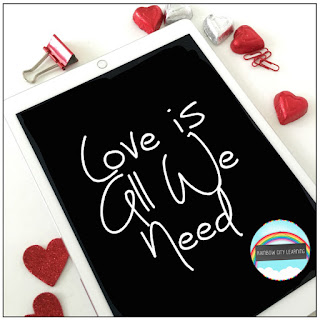Just a little inspiration for building confidence and acceptance in your learning community.

<<<<<<<<<<<<<<<<<<<<<<<<<<<<<<<<<<<<<<<<<<<<<<<<<<<<<<<<<<<

IMWAYR: Funny Bones

By Lisa Robles of LisaTeachR’s ClassroomHave you read Funny Bones? Winner of multiple awards and a great informational addition to your Dia de Los Muertos collection!

<<<<<<<<<<<<<<<<<<<<<<<<<<<<<<<<<<<<<<<<<<<<<<<<<<<<<<<<<<<

Valentine’s Day STEM Olympics

By Kerry Tracy of Kerry TracyBlog post outlines 5 infatuating Valentine’s Day-themed STEM challenges that can be modified for use with grades 2-8. Help Cupid get some target practice, build a tower of love, design the perfect candy container, find the “heaviest” heart, and have a flower frenzy!

<<<<<<<<<<<<<<<<<<<<<<<<<<<<<<<<<<<<<<<<<<<<<<<<<<<<<<<<<<<

Cooperative Learning – Bring Core Subjects Together for Student Learning!

By M. Moore of Moore ResourcesCooperative Learning – Bring Core Subjects Together for Student Learning!

<<<<<<<<<<<<<<<<<<<<<<<<<<<<<<<<<<<<<<<<<<<<<<<<<<<<<<<<<<<

What Word Does This Say: B-L-E-N-D?

By Susan Berkowitz of Susan BerkowitzThis is part of a series of posts about phonological awareness and the different steps and skills to teach. This post is about blending sounds and syllables to hear the word.

<<<<<<<<<<<<<<<<<<<<<<<<<<<<<<<<<<<<<<<<<<<<<<<<<<<<<<<<<<<

Teaching Self-Regulation Skills to Elementary Age Children

By Thia Triggs of Print PathFinally! A systematic method to teach self-regulation skills to children with sensory, emotional, and behavioral needs. Color coded task cards give students managed choices to learn specific skills that meet their sensory needs and also calm them down, so that they can perform their academic classroom tasks.

<<<<<<<<<<<<<<<<<<<<<<<<<<<<<<<<<<<<<<<<<<<<<<<<<<<<<<<<<<<

My Funny Valentine: Love Letters by Arnold Adoff

By Tracy Willis of Wild Child Designs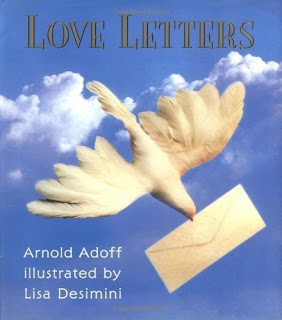A review and glimpse into “Love Letters” poetry by Arnold Adoff. This post also includes a teaching idea for its use in the classroom.

<<<<<<<<<<<<<<<<<<<<<<<<<<<<<<<<<<<<<<<<<<<<<<<<<<<<<<<<<<<

Robots + Math & Science = Total Engagement

By Megan Bodmann of Adventures Teaching 4thGet your students engaged in learning by introducing robots into your classroom. They are not only a ton of fun, but you can easily utilize them in your math and science lessons. Find out how easy it is to do!

<<<<<<<<<<<<<<<<<<<<<<<<<<<<<<<<<<<<<<<<<<<<<<<<<<<<<<<<<<<

Playground Problem = Real Life

By Kathie Yonemura of Tried and True Teaching Tools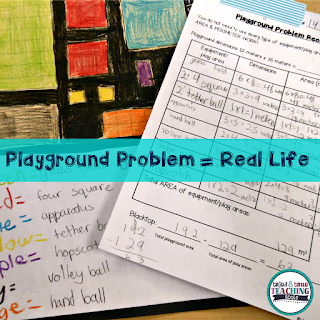The Playground Problem is a real-life math challenge! It keep students engaged and practicing their area and perimeter skills in a meaningful way.

<<<<<<<<<<<<<<<<<<<<<<<<<<<<<<<<<<<<<<<<<<<<<<<<<<<<<<<<<<<

Understanding Fractions: A 6-Part Series

By Shametria Routt of The Routty Math TeacherFractions– a single word that deflates the confidence of our most competent students and adults alike. In this series, I share some of the essential fraction understandings that I have developed over the years, including the many math tools you can use to reinforce these essential skills. With that in mind, each of the six posts highlights a fraction tool and activity that can be used to address a specific Common Core Math Standard (with a few printable freebies too).

<<<<<<<<<<<<<<<<<<<<<<<<<<<<<<<<<<<<<<<<<<<<<<<<<<<<<<<<<<<

Leveled Math Assessments

By Tammy Roose of Tarheel State Teacher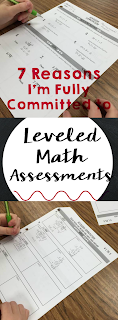Do your math assessments encourage a growth mindset? Create an entry point for students who are not yet meeting the standards? Allow above average learners to show they know more than just what’s expected for their grade-level? Find out 7 reasons why I’m committed to leveled math assessments this year!

<<<<<<<<<<<<<<<<<<<<<<<<<<<<<<<<<<<<<<<<<<<<<<<<<<<<<<<<<<<

Here’s your chance to hop on over and visit the blog posts of our creative teachers.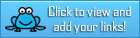## FREE LANGUAGE ARTS LESSON – “Literacy Worksheets for Any Book”

by TchrBrowne4 literacy worksheets that can be used with any picture book.

If you like these and would want more you can visit by store and see my package of 21 literacy worksheets for any book plus a list of recommended picture books.
21LiteracyWorksheetsForAnyBook

https://www.teacherspayteachers.com/Product/Literacy-Worksheets-for-any-book-95724

Join The Best of Teacher Entrepreneurs Marketing Cooperative at
http://www.thebestofteacherentrepreneursmarketingcooperative.com/2014/01/the-best-of-teacher-entrepreneurs.html
and get THOUSANDS OF PAGE VIEWS for your TpT products!

Go to http://www.pinterest.com/TheBestofTPT/ for even more free products!